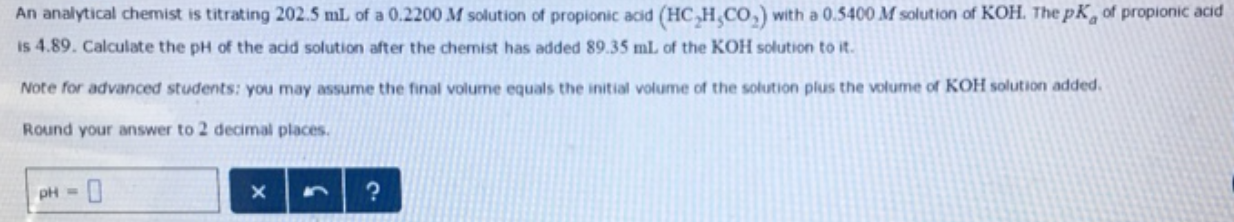# Problem: An analytical chemist is titrating 202.5 mL of a 0.2200 M solution of propionic acid (HC2H5CO2) with a 0.5400 M solution of KOH. The pK a of propionic acid is 4.89. Calculate the pH of the acid solution after the chemist has added 89.35 mL of the KOH solution to it. Note for advanced students: you may assume the final volume equals the initial volume of the solution plus the volume of KOH solution added. Round your answer to 2 decimal places.

###### FREE Expert Solution
87% (95 ratings)###### Problem Details

An analytical chemist is titrating 202.5 mL of a 0.2200 M solution of propionic acid (HC2H5CO2) with a 0.5400 M solution of KOH. The pK a of propionic acid is 4.89. Calculate the pH of the acid solution after the chemist has added 89.35 mL of the KOH solution to it.

Note for advanced students: you may assume the final volume equals the initial volume of the solution plus the volume of KOH solution added. Round your answer to 2 decimal places.